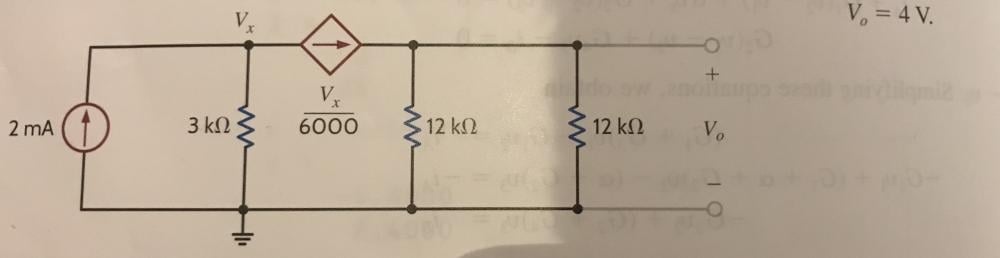# Problem with node analysis

Can someone explain to me what i'm doing wrong?

Vo = 4V- 2  + ( Vx / 3k ) + ( Vx / 6000 ) = 0

12000  = - 2000Vx  - Vx

12000 / - 2001 = Vx

Vx = ~ 6vSimple solution -

The driving capability of the current source is 2mA.

The at the Vx point the current should be = (vx/3000Ohms) + (vx/6000)

Since the current driving source can not be more than 2mA,

0.002A = (vx/3000Ohms)A + (vx/6000)A

Vx = 4V.

Since it is the same node Vx = Vout.

Joined February 12, 2018      696
Monday at 02:11 PMThanks for helping

Joined July 11, 2021      1
Sunday at 12:16 AM